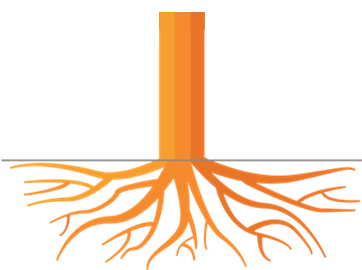# Regression

### What is Multicollinearity? A Visual Description

November 20th, 2019 byMulticollinearity is one of those terms in statistics that is often defined in one of two ways:

1. Very mathematical terms that make no sense — I mean, what is a linear combination anyway?

2. Completely oversimplified in order to avoid the mathematical terms — it’s a high correlation, right?

So what is it really? In English?

### Member Training: Elements of Experimental Design

August 1st, 2019 byWhether or not you run experiments, there are elements of experimental design that affect how you need to analyze many types of studies.

The most fundamental of these are replication, randomization, and blocking. These key design elements come up in studies under all sorts of names: trials, replicates, multi-level nesting, repeated measures. Any data set that requires mixed or multilevel models has some of these design elements. (more…)

### Member Training: A Predictive Modeling Primer: Regression and Beyond

May 31st, 2019 byPredicting future outcomes, the next steps in a process, or the best choice(s) from an array of possibilities are all essential needs in many fields. The predictive model is used as a decision making tool in advertising and marketing, meteorology, economics, insurance, health care, engineering, and would probably be useful in your work too! (more…)

### Statistical Models for Truncated and Censored Data

November 12th, 2018 by

by Jeff Meyer

As mentioned in a previous post, there is a significant difference between truncated and censored data.

Truncated data eliminates observations from an analysis based on a maximum and/or minimum value for a variable.

Censored data has limits on the maximum and/or minimum value for a variable but includes all observations in the analysis.

As a result, the models for analysis of these data are different. (more…)

### The Four Stages of Statistical Skill

September 21st, 2018 by

At The Analysis Factor, we are on a mission to help researchers improve their statistical skills so they can do amazing research.

We all tend to think of “Statistical Analysis” as one big skill, but it’s not.

Over the years of training, coaching, and mentoring data analysts at all stages, I’ve realized there are four fundamental stages of statistical skill:Stage 1: The FundamentalsStage 2: Linear ModelsStage 3: Extensions of Linear ModelsThere is also a stage beyond these where the mathematical statisticians dwell. But that stage is required for such a tiny fraction of data analysis projects, we’re going to ignore that one for now.

If you try to master the skill of “statistical analysis” as a whole, it’s going to be overwhelming.

And honestly, you’ll never finish. It’s too big of a field.

But if you can work through these stages, you’ll find you can learn and do just about any statistical analysis you need to. (more…)

### Member Training: Quantile Regression: Going Beyond the Mean

September 1st, 2017 by

In your typical statistical work, chances are you have already used quantiles such as the median, 25th or 75th percentiles as descriptive statistics.But did you know quantiles are also valuable in regression, where they can answer a broader set of research questions than standard linear regression?

In standard linear regression, the focus is on estimating the mean of a response variable given a set of predictor variables.

In quantile regression, we can go beyond the mean of the response variable. Instead we can understand how predictor variables predict (1) the entire distribution of the response variable or (2) one or more relevant features (e.g., center, spread, shape) of this distribution.

For example, quantile regression can help us understand not only how age predicts the mean or median income, but also how age predicts the 75th or 25th percentile of the income distribution.

Or we can see how the inter-quartile range — the width between the 75th and 25th percentile — is affected by age. Perhaps the range becomes wider as age increases, signaling that an increase in age is associated with an increase in income variability.

In this webinar, we will help you become familiar with the power and versatility of quantile regression by discussing topics such as:

• Quantiles – a brief review of their computation, interpretation and uses;
• Distinction between conditional and unconditional quantiles;
• Formulation and estimation of conditional quantile regression models;
• Interpretation of results produced by conditional quantile regression models;
• Graphical displays for visualizing the results of conditional quantile regression models;
• Inference and prediction for conditional quantile regression models;
• Software options for fitting quantile regression models.

Join us on this webinar to understand how quantile regression can be used to expand the scope of research questions you can address with your data.

Note: This training is an exclusive benefit to members of the Statistically Speaking Membership Program and part of the Stat’s Amore Trainings Series. Each Stat’s Amore Training is approximately 90 minutes long.